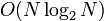# Generating set-based black-box group algorithm for abelianness testing

## Summary

Item Value
Problem being solved abelianness testing problem: determine whether a given group is abelian
Input format a finite group$G$ given via an encoding of a group
Output format Yes/No depending on whether$G$ is abelian
In the No case it is also possible to output a pair of elements that do not commute.
Theoretical significance This shows that the abelianness testing problem is in$P$ by explicitly finding a polynomial-time algorithm for it.
Running time$O(N\log_2^2N)$ times the time taken for group operations (multiplication, equality checking).
Competing algorithms brute-force black-box group algorithm for abelianness testing: This is slower, but much easier to code and also much easier to parallelize. It checks all pairs of elements
nondeterministic black-box group algorithm for abelianness testing: nondeterministic algorithm that first guesses a generating set, then verifies that it generates the whole group and that the elements in the generating set commute with each other. Takes time$O(N\log_2N)$ times the time taken for group operations.
randomized black-box group algorithm for abelianness testing: Takes time$O(\log_2N)$ plus the time for group operations, assuming that lookup of random elements takes logarithmic time. The idea is to randomly select pairs of elements in the group and check whether they commute. A No answer means the group is definitely non-abelian, and a Yes answer means it is probably abelian, with the trade-off between probability and the$O$-constant independent of$N$.

## Idea and outline

The algorithm combines two algorithms:

1. It first finds a generating set of size at most$\log_2N$ using the black-box group algorithm for small generating set-finding problem. The time taken for this is$O(N\log_2^2N)$ times the time for group operations.
2. It then applies the black-box group algorithm for abelianness testing given a generating set.